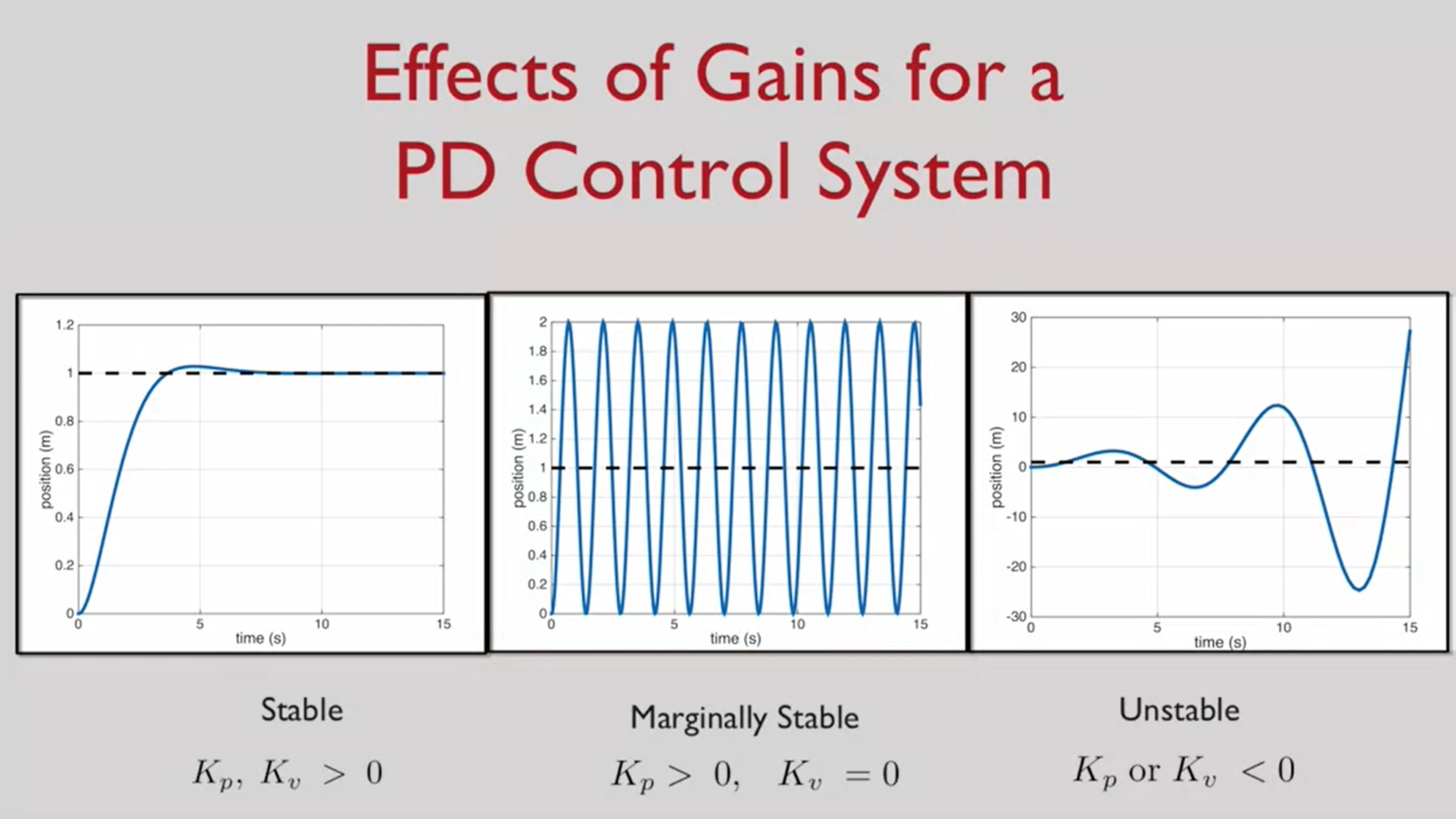# Robotics-Flight week 1 and 2

less than 1 minute read

## 准备看点Coursera课程

• Coursera: robotics-flight
• Week 1
• State Estimation
• obtaub reliable estimates of position and velocity
• SLAM(Simultaneous Localization And Mapping)
• Control for trajectory tracking in a simple second-order system
• PD control
• $u(t) = x’’^{des}(t)+K_ve’(t)+K_pe(t)$
• PID control (proportion integration differentiation)
• $u(t) = x’’^{des}(t)+K_ve’(t)+K_pe(t)+K_i\int_0^t{e(\tau)d\tau}$
•• code Here
•• Week2
• rotation matrix
• is orthogonal matrix
• all vector is 1
• $R^{-1} = R^T$

## Ubuntu chromedriver remote browser

location /chrome/ {
proxy_pass http://127.0.0.1:9222/;
proxy_http_version 1.1;
proxy_set_header Host "[********]";  # cause chrome use host as ws address
sub_filter '/json' '/chrome/json';
sub_filter_types *;
sub_filter_once off;
}


## what is the next

• This request has been blocked; this endpoint must be available over WSS.

Categories:

Updated: